# Finding Duplicate Elements in an Unsorted Array in C

## Finding Duplicate Elements in an Unsorted Array in C Language:

In this article, I am going to discuss Finding Duplicate Elements in an Unsorted Array in C Language with Examples. In our previous article, where we discussed Finding Duplicate Elements in a Sorted Array using Hashing in C Language.

##### How to Find Duplicate Elements in an Unsorted Array?

We have taken an array where elements are not sorted and contain duplicate elements i.e. 8, 6. Now we have to write a procedure to find out duplicate elements and also we want to count those duplicate elements. So, there can be more than one solution to find duplicate elements. Let’s see some of the methods: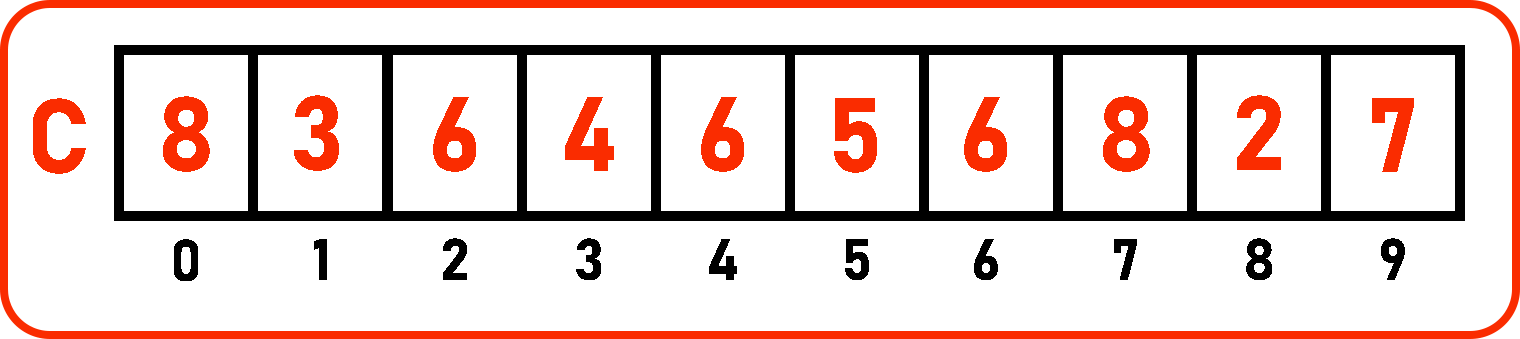##### 1st Method to Find Duplicate Elements in an Unsorted Array:

In this method, we will scan through an array, pick an element and look for its duplicate. Let’s take an example of 8, we will search through the above array and check if there is 8’s duplicate element presented in the array.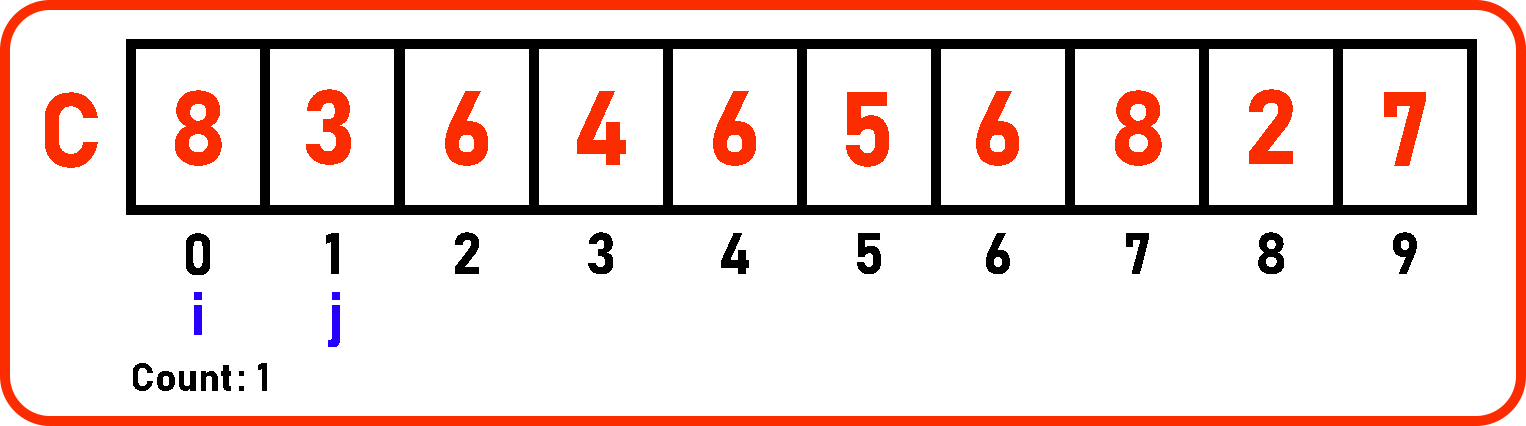Here we are taking a variable of count which will count how many times a particular element is appearing in the array. i will point to the element which we want to find the duplicate in the given array and j will search the element in the array and if it found the same element we have to increment the count variable. Here i is pointing to the 0th index.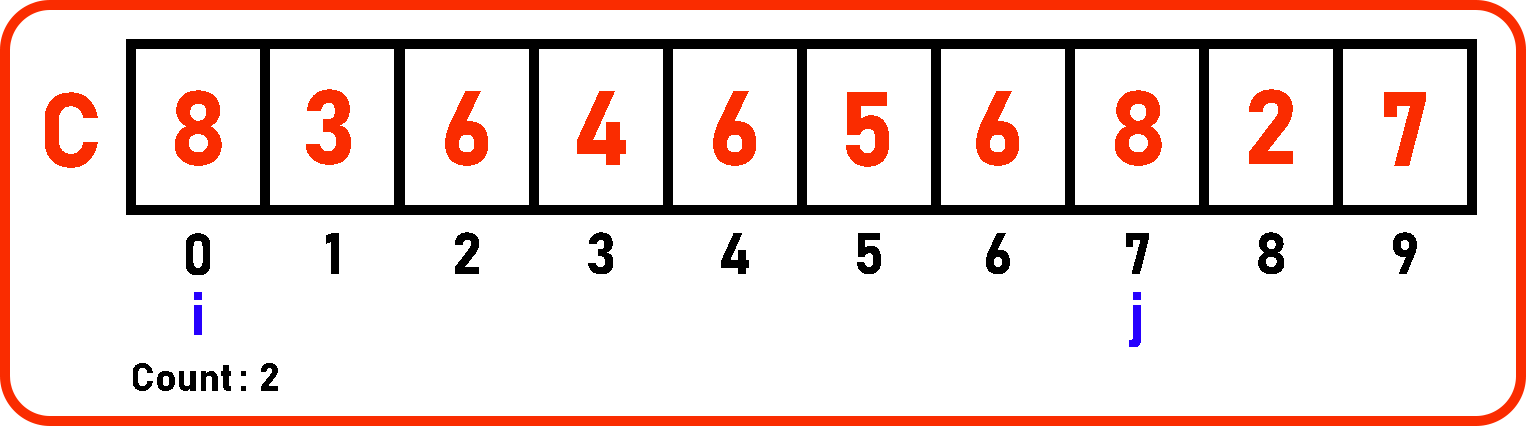Now, i is pointing to the 7th index where i found a duplicate element of 8. So, here we have to increment the count to 2. Here we have to consider one thing, when we found another copy of any element, we have changed that to -1, it may happen that the same element is counted multiple times, so we don’t want to count that value again. We have some marking so that we wouldn’t consider it again. So here we modified the 8 to -1: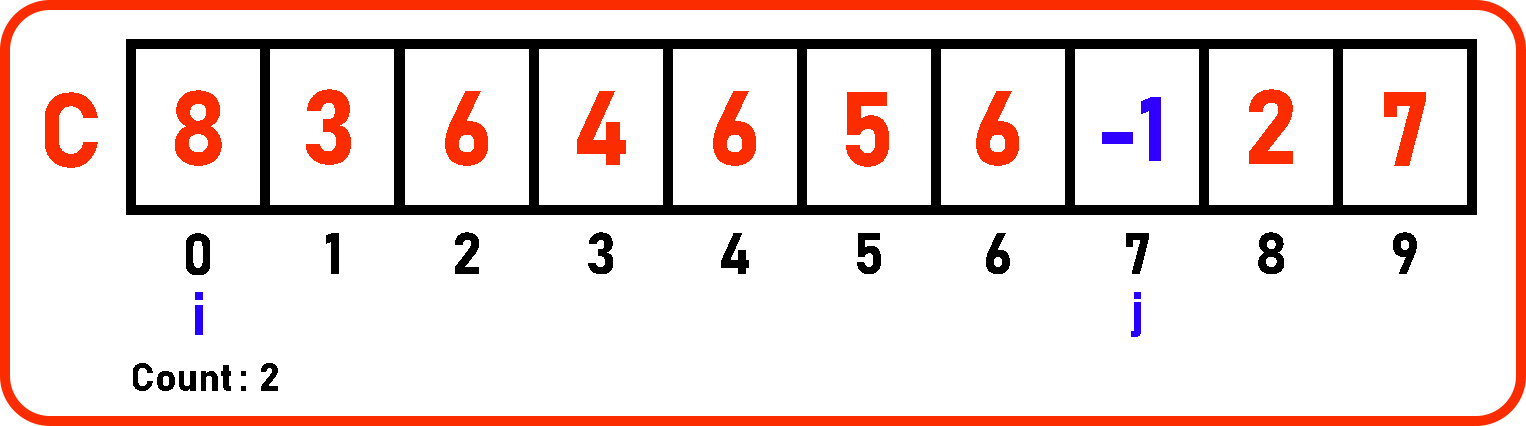-1 means the element is not there. So, here we have finished looking for the duplicate of 8. The value of the count is 2 for element 8. Now, let’s continue, we will find the duplicates of other elements. So, as we counted all duplicates of 8, now increment i to next element and j to i+1 position: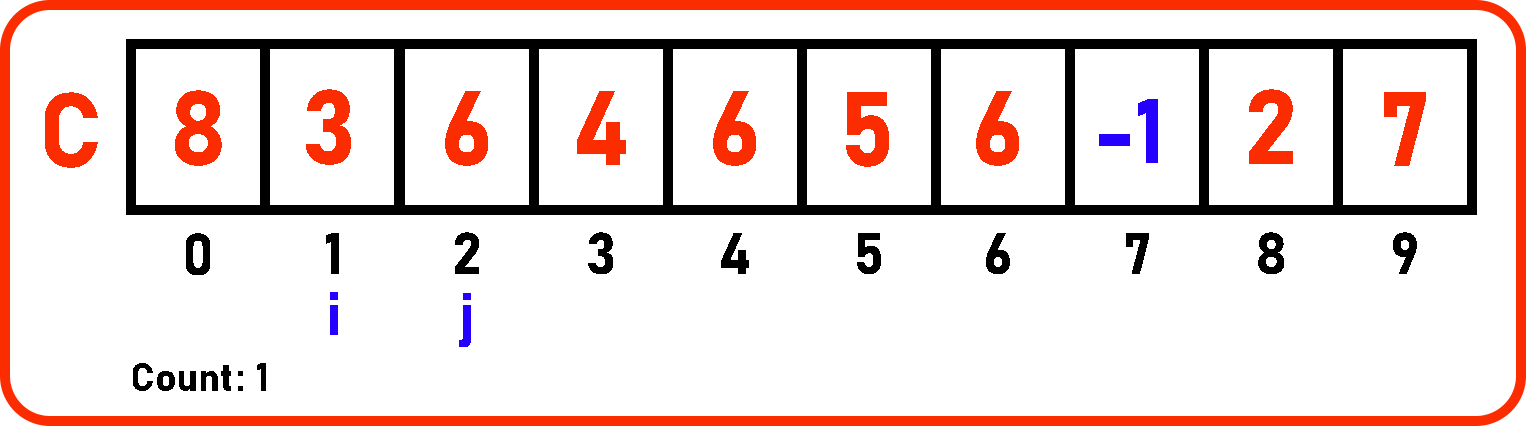Here i is pointing on 3. As we can see in the above array, there is no duplicate of element 3 so j will trace the array from the i+1 position. Here there is no increment in the value of count as we haven’t found any duplicate of 3. So points to the next element: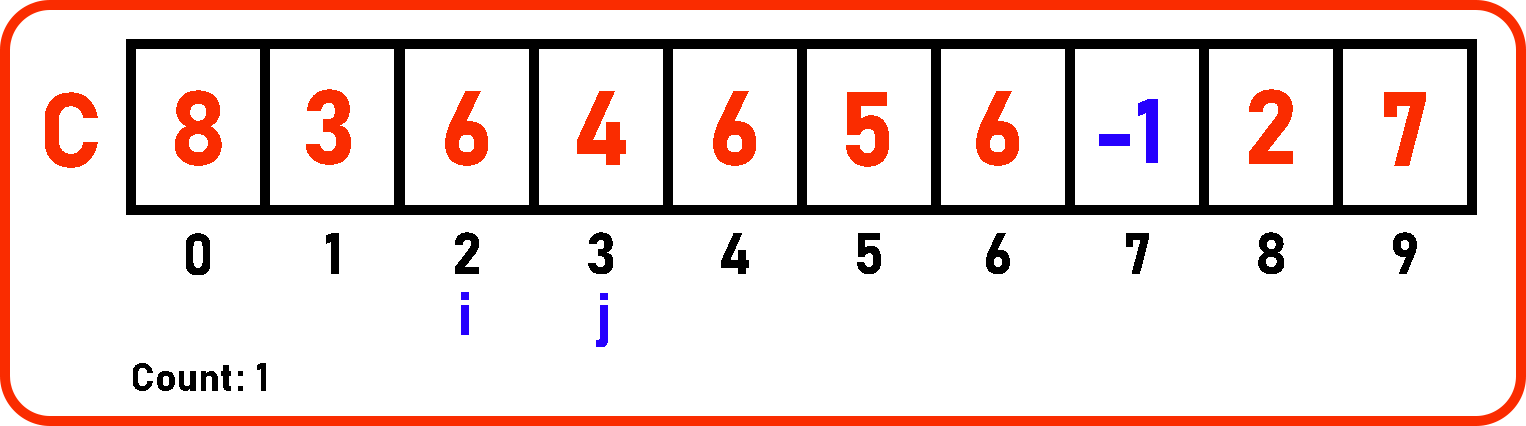Now i is pointing to the 6 elements and j is pointing to the i+1 position. The value of count is 1.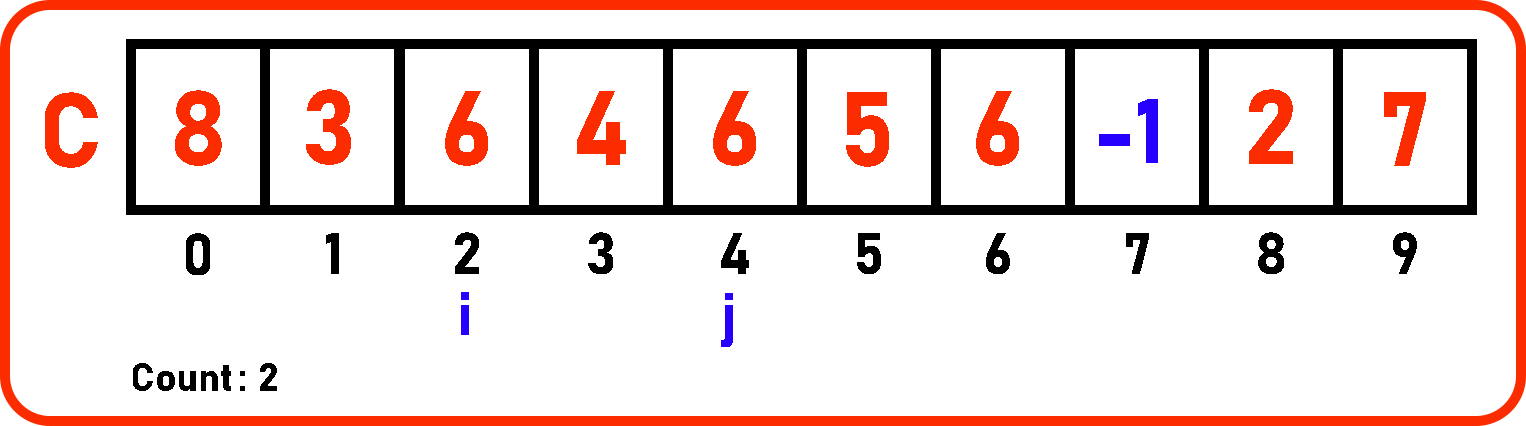Now j is pointing to the duplicate of 6 elements. So here we have incremented count as 2. Also, we have to make this -1 so that it will not count again: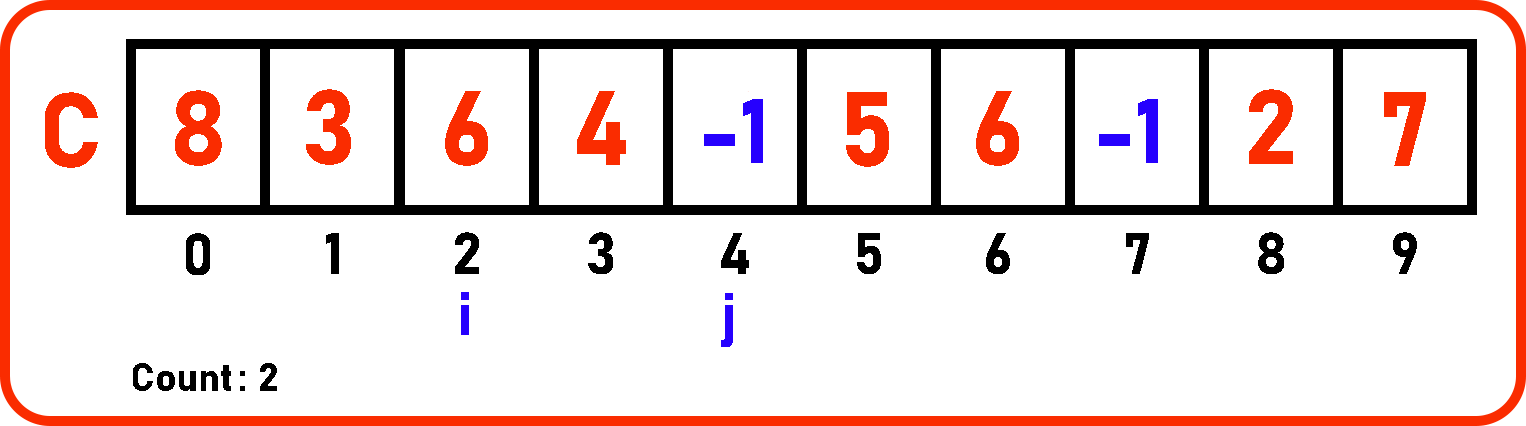Here we removed that duplicate element and mark it as -1. Now j will point next duplicate element of 6 as: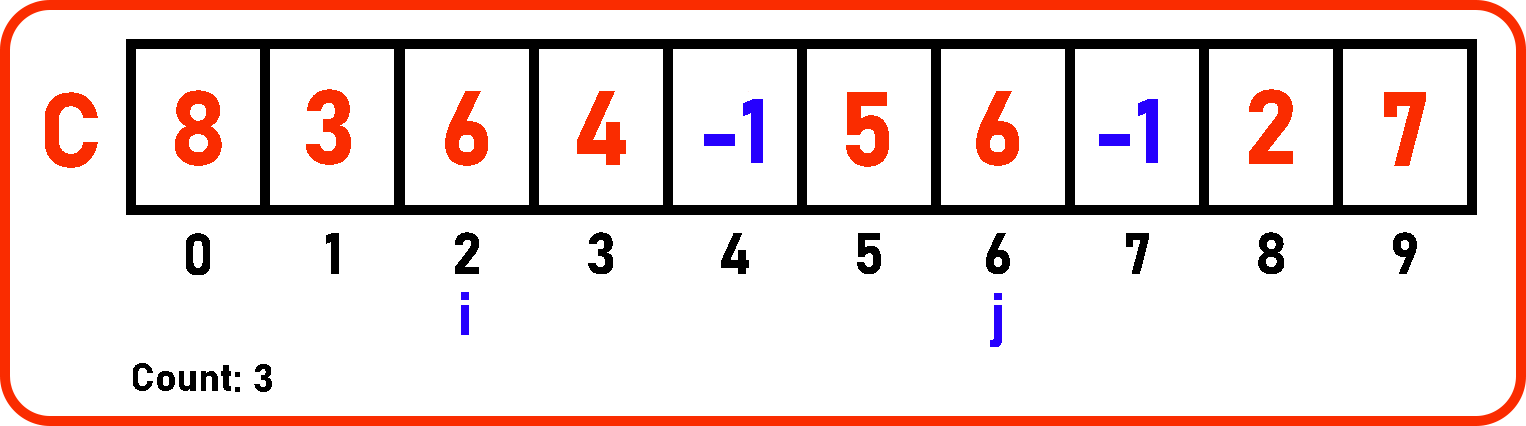Here again, we have found a duplicate element of 6, so we incremented the count.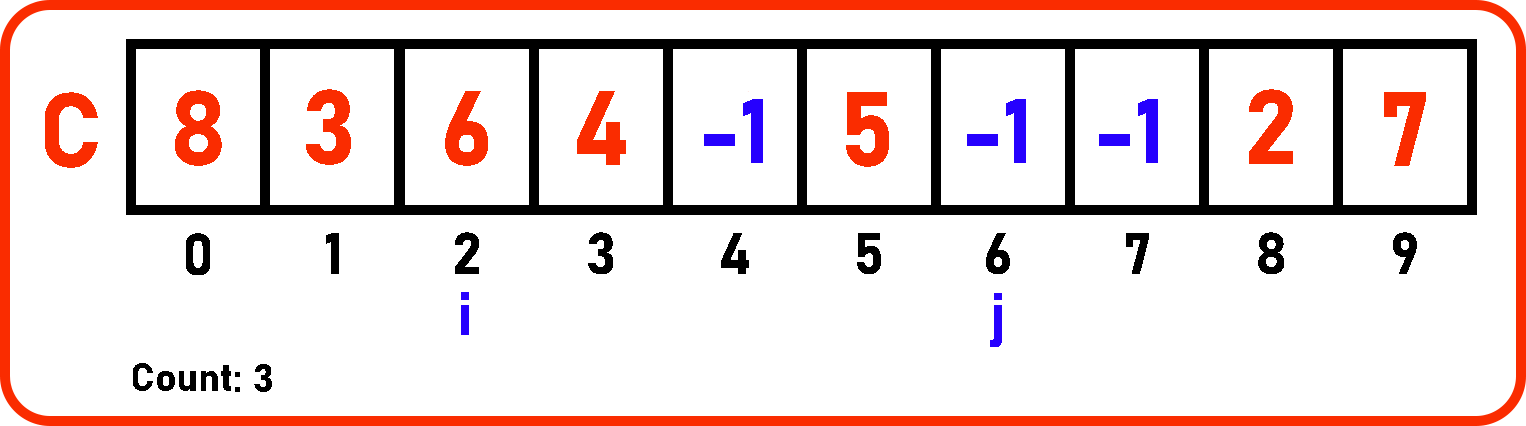Here we marked that duplicate element as -1.

##### Time Complexity:

Let’s do some analysis: For finding duplicates, I am comparing them with the rest of the elements. So if there are total n elements then we are comparing as:
= n-1 + n-2 + n-3 + … + 3 + 2 + 1.
We can write the above series as:
= n (n-1) / 2
= (n2 – n) / 2

Time Complexity: O (n)

##### Finding Duplicate Elements in an Unsorted Array Code in C Language:
```#include <stdio.h>
#include <stdlib.h>

struct List{
int C;
int size;
int length;
};

void Display(struct List list) {
int i;
printf("Elements are:\n");
for (i = 0;i<list.length;i++)
printf("%d ", list.C[i]);
printf("\n\n");
}

void DuplicateUnsorted(struct List* list){

for(int i = 0; i <= list->length - 1; i++){

int count = 1;

for(int j = i+1; j < list->length; j++){
if(list->C[i] == list->C[j]){
count++;
list->C[j] = -1;
}
}
if(count > 1 && list->C[i] != -1){
printf("%d is appearing: %d times\n", list->C[i], count);
}
}
}

int main(){
struct List list_1 = {{8, 3, 6, 4, 6, 5, 6, 8, 2, 7}, 10, 10};
Display(list_1);
DuplicateUnsorted(&list_1);
}
```
###### Output: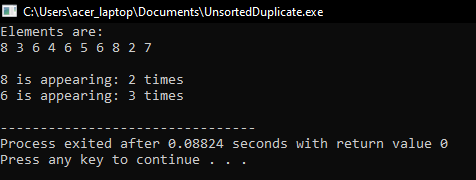##### 2nd Method to Find Duplicate Elements in an Unsorted Array:

Now, let’s see another method for finding and counting duplicate elements in an unsorted array. In this method, we are using a hash table. We discussed the hash table in previous articles.Here also we are taking the same example which we discussed in 1st method.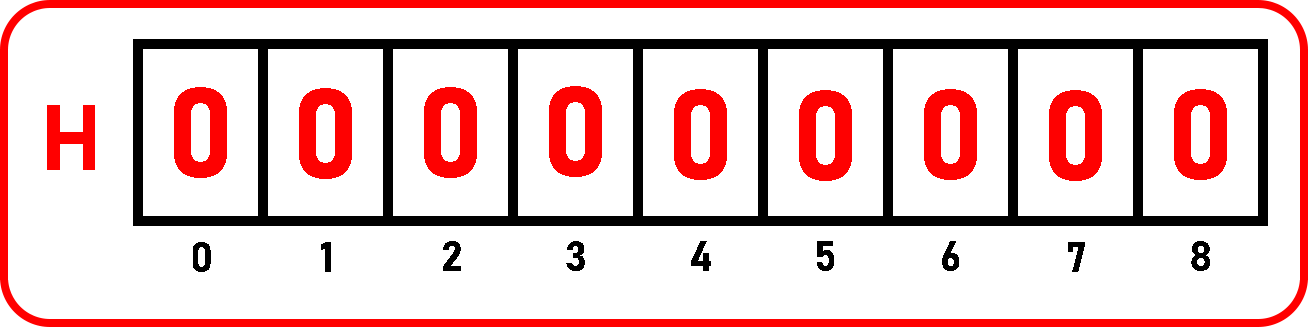Above is our hash table or extra array for storing the occurrence of each and every element of the given array. As you can see all indices contain zero in the hash table. As we scan through the given array, we will increment that particular index of the hash table. The size of the hash table is equal to the largest element of the given element.

The procedure is we have to scan through the list and we have to increment the count in a particular index in the hash table. We will take i variable which will point every element one by one in the given array.

##### 0th Index: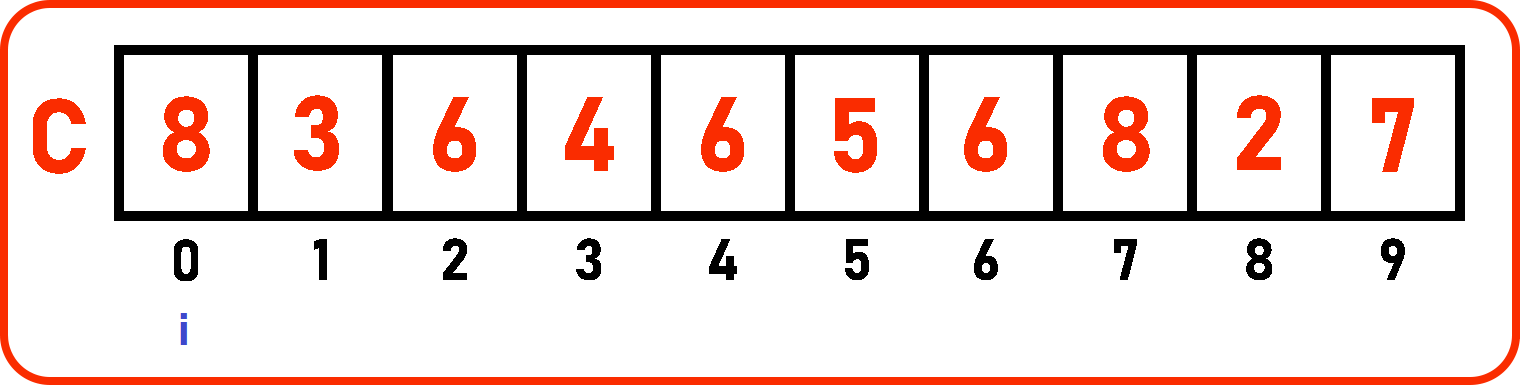Here i is pointing 0th index which has the value of 8. So, we have to increment the 8th index in the hash table: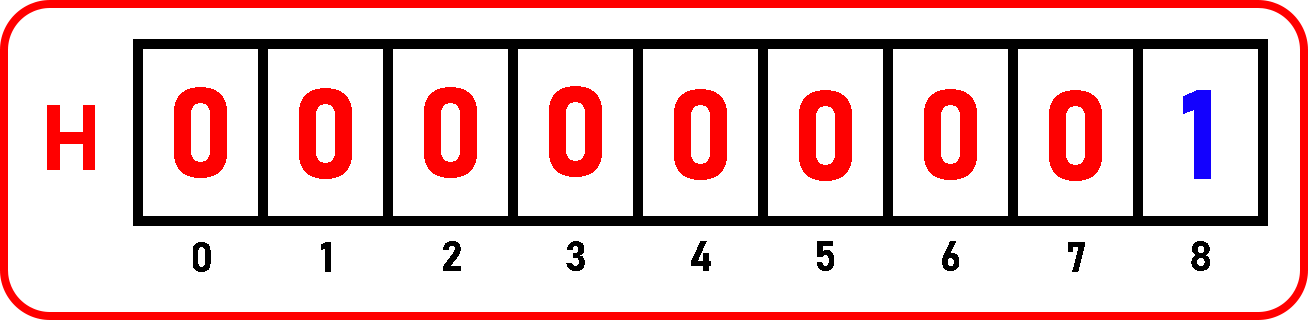##### 1st Index: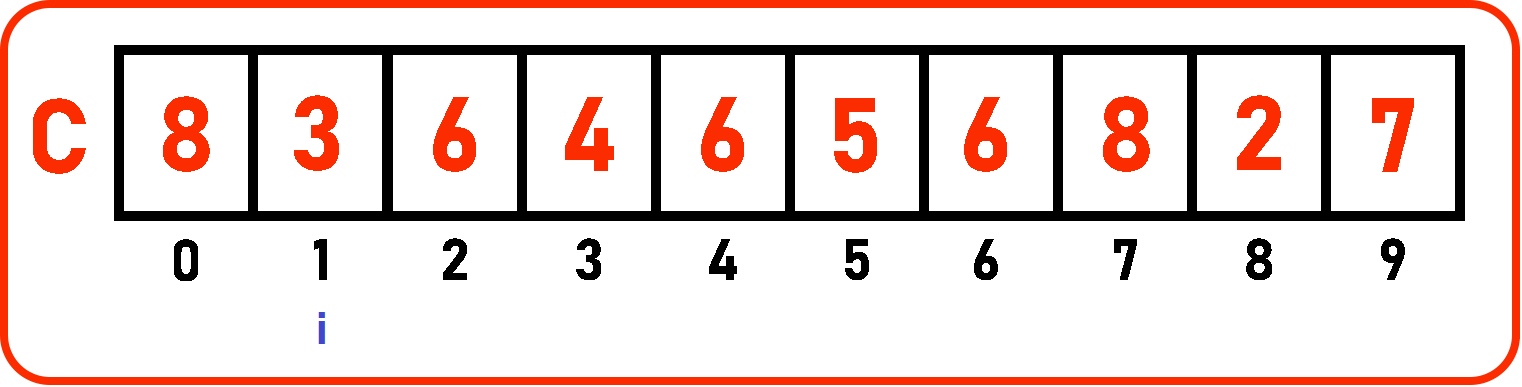Now, i is pointing next index which has the value of 3. So increment the value of index 3 in the hash table: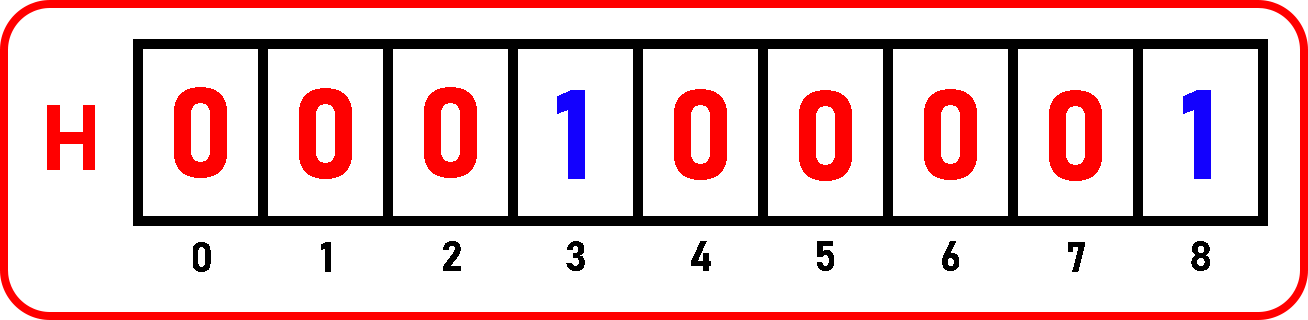##### 2nd Index: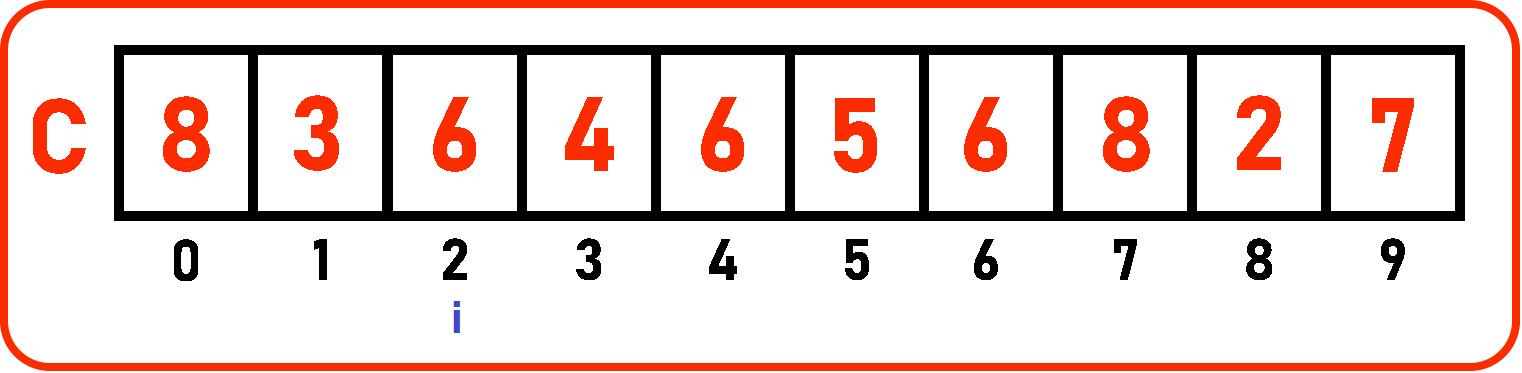Now, i pointing to the 2nd index which has the value of 6. So, increment the value of the 6th index in hast table: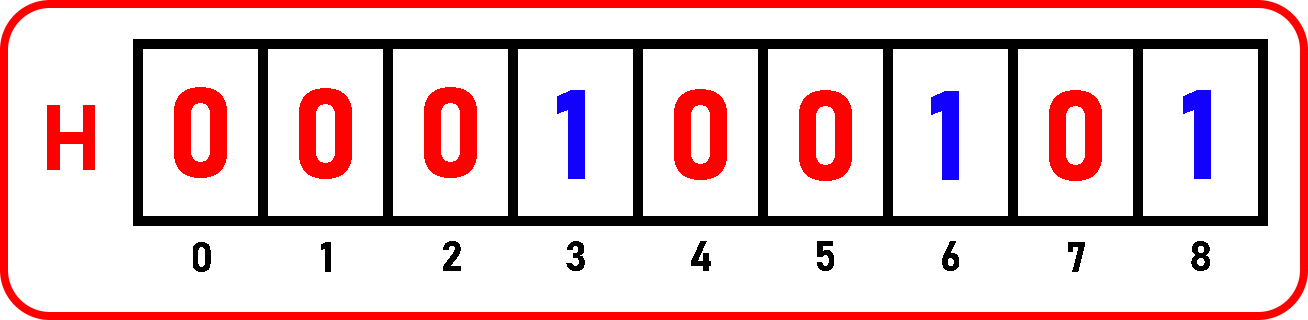In this manner, we will scan through the array with the variable i and increment the count in the hash table, so the final hash table will be: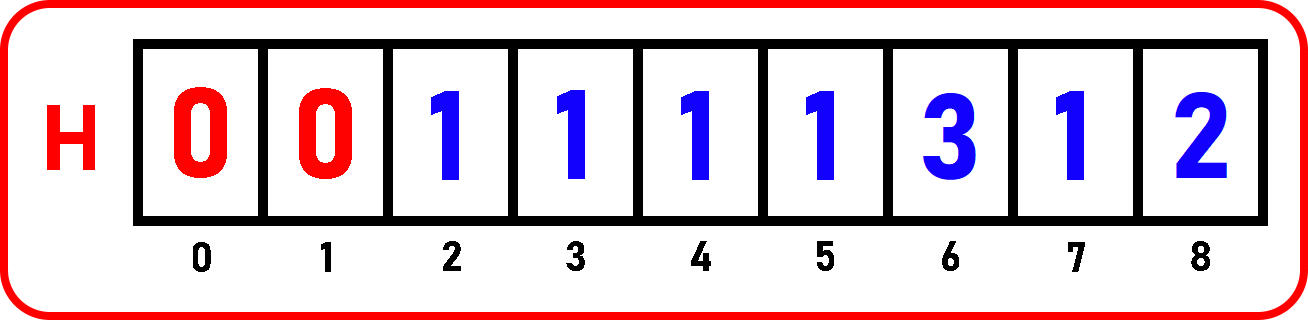The element of the hash table represents the count of the corresponding index in the given array.

Time Complexity: O (n)

##### Finding Duplicate Elements in an Unsorted Array Code in C language:
```#include <stdio.h>
#include <stdlib.h>

struct List{
int C;
int size;
int length;
};

void Display(struct List list) {
int i;
printf("Elements are:\n");
for (i = 0;i<list.length;i++)
printf("%d ", list.C[i]);
printf("\n\n");
}

void DuplicateUnsorted(struct List list, int max){

int H[max];

for(int i = 0; i <= max; i++){
H[i] = 0;
}

for(int i = 0; i < list.length; i++){
H[list.C[i]]++;
}

printf("Duplicate Elements are: \n");
for (int i = 1; i <= max; i++){
if(H[i] > 1)
printf("%d is appearing %d\n", i, H[i]);
}
}

int main(){
struct List list_1 = {{8, 3, 6, 4, 6, 5, 6, 8, 2, 7}, 10, 10};
Display(list_1);
DuplicateUnsorted(list_1, 8);
}
```
###### Output: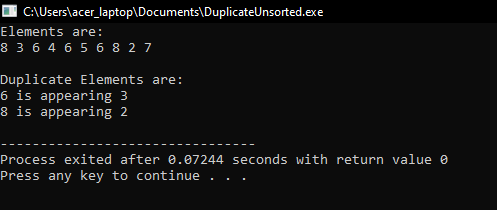In the next article, I am going to discuss How to Find a Pair of Elements with Sum K from an Array in C Language with Examples. Here, in this article, I try to explain How to Find Duplicate Elements in an Unsorted Array in C Language with Examples and I hope you enjoy this Finding Duplicate Elements in an Unsorted Array in C Language with Examples article.